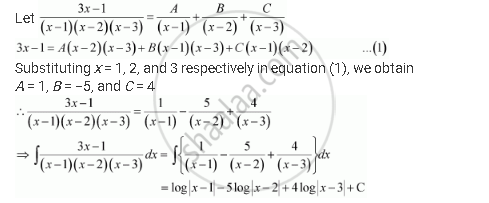Share

# Integrate the Rational Functions (3x - 1)/((X - 1)(X - 2)(X - 3)) - Mathematics

#### Question

Integrate the rational functions (3x - 1)/((x - 1)(x - 2)(x - 3))

#### SolutionIs there an error in this question or solution?

#### Video TutorialsVIEW ALL 

Solution Integrate the Rational Functions (3x - 1)/((X - 1)(X - 2)(X - 3)) Concept: Methods of Integration - Integration Using Partial Fractions.
S